## ML Aggarwal Class 8 Solutions for ICSE Maths Chapter 8 Simple and Compound Interest Ex 8.3

Question 1.
Calculate the amount and compound interest on
(i) ₹15000 for 2 years at 10% per annum compounded annually.
(ii) ₹156250 for $$1 \frac{1}{2}$$ years at 8% per annum compounded half-yearly.
(iii) ₹ 100000 for 9 months at 4% per annum compounded quarterly.
Solution: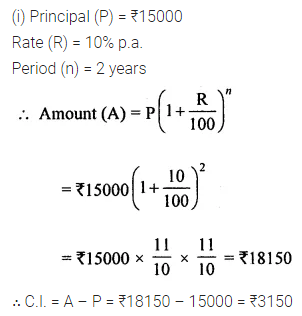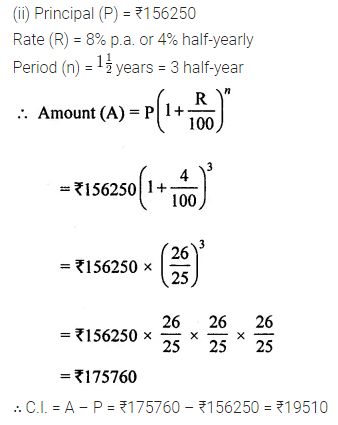Question 2.
Find the difference between the simple interest and compound interest on ₹4800 for 2 years at 5% per annum, compound interest being reckoned annually.
Solution:Question 3.
Find the compound interest on ₹3125 for 3 years if the rates of interest for the first, second and third year are respectively 4%, 5% and 6% per annum.
Solution: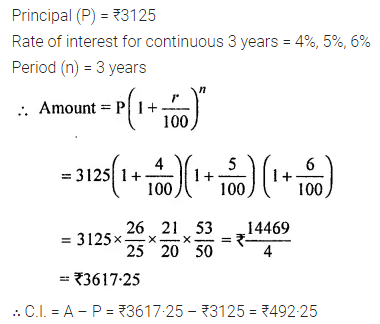Question 4.
Kamla borrowed ₹26400 from a Bank to buy a scooter at a rate of 15% p.a. compounded yearly. What amount will she pay at the end of 2 years and 4 months to clear the loan?
Solution: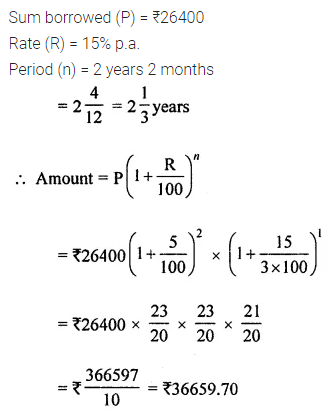Question 5.
Anil borrowed ₹18000 from Rakesh at 8% per annum simple interest for 2 years. If Anil had borrowed this sum at 8% per annum compound interest, what extra amount would he has to pay?
Solution: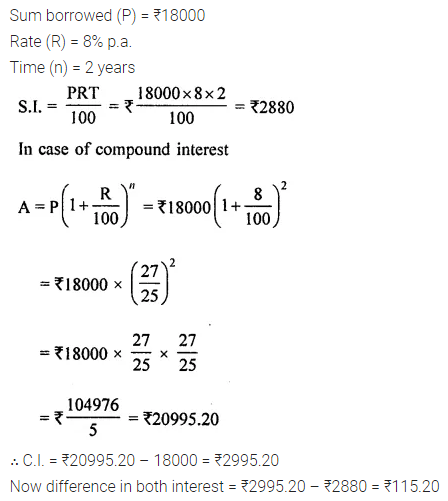Question 6.
Mukesh borrowed 775000 from a bank. If the rate of interest is 12% per annum, find the amount he would be paying after $$1 \frac{1}{2}$$ years if the interest is
(i) compounded annually
(ii) compounded half-yearly.
Solution:Question 7.
Aryaman invested ₹10000 in a company, he would be paid interest at 7% per annum compounded annually. Find
(i) the amount received by him at the end of 2 years.
(ii) the interest for the 3rd year.
Solution:Question 8.
What sum of money will amount to ₹9261 in 3 years at 5% per annum compound interest?
Solution: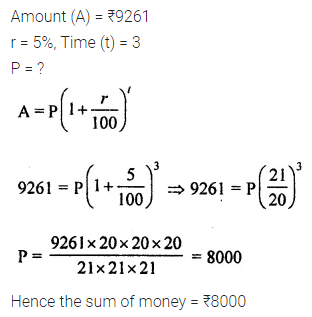Question 9.
What sum invested for $$1 \frac{1}{2}$$ years compounded half-yearly at the rate 8% p.a. will amount to ₹ 140608?
Solution:Question 10.
At what rate percent will ₹2000 amount to ₹2315·25 in 3 years at compound interest?
Solution: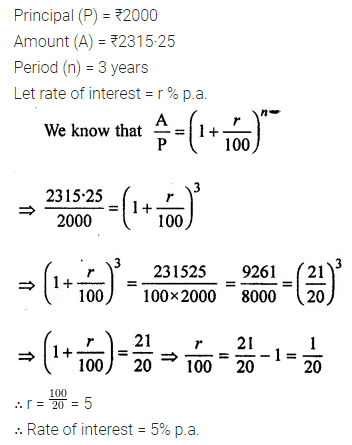Question 11.
If ₹40000 amounts to ₹46305 in $$1 \frac{1}{2}$$ years, compound interest payable half-yearly, find the rate of interest per annum.
Solution: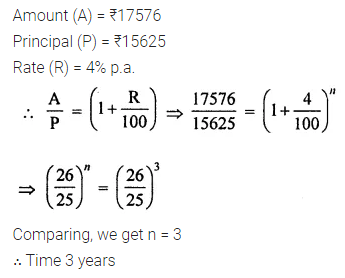Question 12.
In what time will ₹15625 amount to ₹ 17576 at 4% per annum compound interest?
Solution: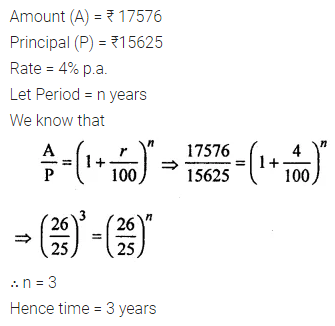Question 13.
₹ 16000 invested at 10% p.a. compounded semi-annually, amounts to ₹18522. Find the time period of investment.
Solution: